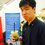# Achieving every integer through mathematical functions

I was offered a problem in which you were given four 9's like this:

9 9 9 9

And by adding mathematical functions (+, -, /, *, floor/ceiling, log, ln, trig, factorial) you could make it sum to the numbers 1-100.

Which made me think: Given a positive integer $n$, how many integers can you achieve from using some sequence of these mathematical functions -- can you get all of them? Only positive integers? I have a feeling that you can get at least every positive integer, but I don't have the evidence to prove it.Note by Michael Tong
6 years, 11 months ago

This discussion board is a place to discuss our Daily Challenges and the math and science related to those challenges. Explanations are more than just a solution — they should explain the steps and thinking strategies that you used to obtain the solution. Comments should further the discussion of math and science.

When posting on Brilliant:

• Use the emojis to react to an explanation, whether you're congratulating a job well done , or just really confused .
• Ask specific questions about the challenge or the steps in somebody's explanation. Well-posed questions can add a lot to the discussion, but posting "I don't understand!" doesn't help anyone.
• Try to contribute something new to the discussion, whether it is an extension, generalization or other idea related to the challenge.

MarkdownAppears as
*italics* or _italics_ italics
**bold** or __bold__ bold
- bulleted- list
• bulleted
• list
1. numbered2. list
1. numbered
2. list
Note: you must add a full line of space before and after lists for them to show up correctly
paragraph 1paragraph 2

paragraph 1

paragraph 2

[example link](https://brilliant.org)example link
> This is a quote
This is a quote
    # I indented these lines
# 4 spaces, and now they show
# up as a code block.

print "hello world"
# I indented these lines
# 4 spaces, and now they show
# up as a code block.

print "hello world"
MathAppears as
Remember to wrap math in $$ ... $$ or $ ... $ to ensure proper formatting.
2 \times 3 $2 \times 3$
2^{34} $2^{34}$
a_{i-1} $a_{i-1}$
\frac{2}{3} $\frac{2}{3}$
\sqrt{2} $\sqrt{2}$
\sum_{i=1}^3 $\sum_{i=1}^3$
\sin \theta $\sin \theta$
\boxed{123} $\boxed{123}$

Sort by:

So, what exactly are we allowed to use? Just one $n$, or $n$ 9's, or something else? Also, do we have to use all the digits we are given?

- 6 years, 11 months ago

Well the introductory problem was just stating motivation for the question. I mean for any positive integer $n$, greater than $1$ I guess (since factorial is the only way to grow). You're allowed to use I guess the functions I stated (since I'm sure there are some crazy functions out there that can easily allow you to get any number).

- 6 years, 11 months ago

Define $\spadesuit(n) = n+1$. Then, $\spadesuit(1) = 2$, $\spadesuit(\spadesuit(1)) = 3$, $\spadesuit(\spadesuit(\spadesuit(1))) = 4$, etc.

So, you don't really need a "crazy" function in order to make every positive integer. This is why providing a list of well defined functions is necessary for this to be a well posed question.

Regardless, what digits and how many of them are we allowed to use? Without any restrictions, I can "make" any number by starting with that number (no functions needed).

- 6 years, 11 months ago

I said to look at the ones I said in the problem... did you miss that part of my post?

And $n$ is an arbitrary integer, not every integer. I think that should be pretty obvious, to be frank.

- 6 years, 11 months ago

As for functions, I did not miss that part of your post. I was merely demonstrating that we don't need a "crazy" function to make every positive integer.

My question was what digits are we allowed to use. The example you have has four 9's. Is that what we are starting with here?

- 6 years, 11 months ago